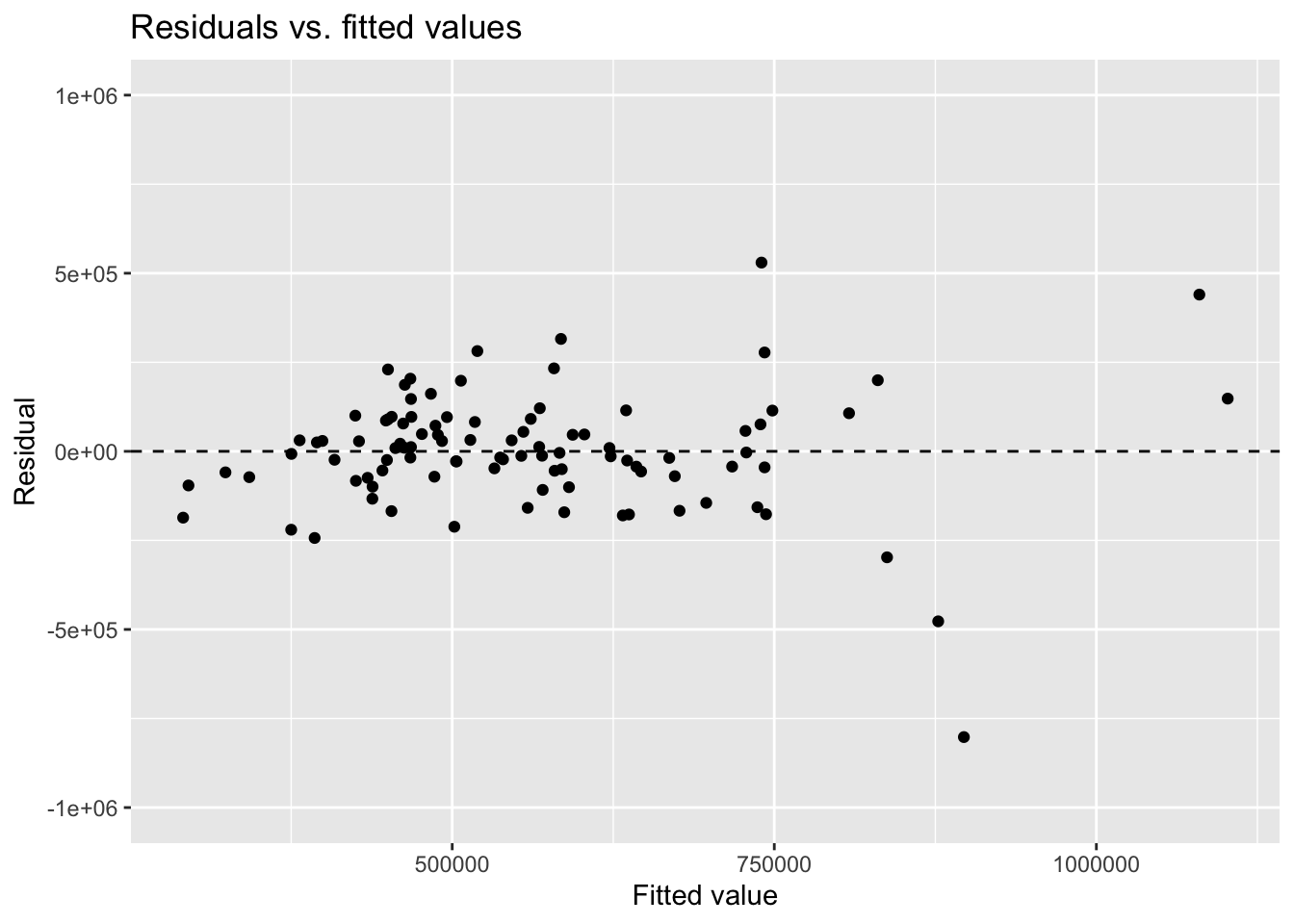Checking model conditions

Important

Go to the course GitHub organization and locate the repo titled `ae-3-duke-forest-YOUR_GITHUB_USERNAME` to get started.

## Packages

``````library(tidyverse)
library(tidymodels)
library(openintro)
library(knitr)``````

## Predict sale price from area

``````df_fit <- linear_reg() %>%
set_engine("lm") %>%
fit(price ~ area, data = duke_forest)

tidy(df_fit) %>%
kable(digits = 2)``````
term estimate std.error statistic p.value
(Intercept) 116652.33 53302.46 2.19 0.03
area 159.48 18.17 8.78 0.00

## Model conditions

### Exercise 1

The following code produces the residuals vs. fitted values plot for this model. Comment out the layer that defines the y-axis limits and re-create the plot. How does the plot change? Why might we want to define the limits explicitly?

``````df_aug <- augment(df_fit\$fit)

ggplot(df_aug, aes(x = .fitted, y = .resid)) +
geom_point() +
geom_hline(yintercept = 0, linetype = "dashed") +
ylim(-1000000, 1000000) +
labs(
x = "Fitted value", y = "Residual",
title = "Residuals vs. fitted values"
)``````### Exercise 2

Improve how the values on the axes of the plot are displayed by modifying the code below.

``````ggplot(df_aug, aes(x = .fitted, y = .resid)) +
geom_point() +
geom_hline(yintercept = 0, linetype = "dashed") +
ylim(-1000000, 1000000) +
labs(
x = "Fitted value", y = "Residual",
title = "Residuals vs. fitted values"
)``````